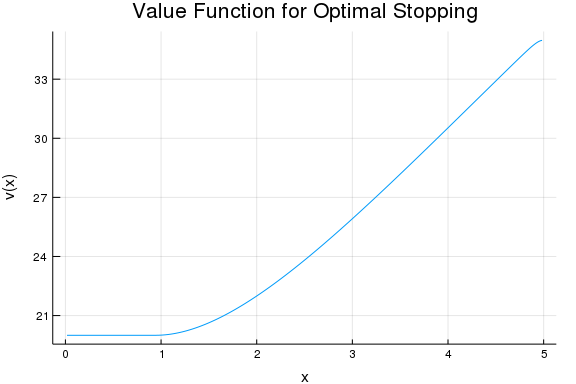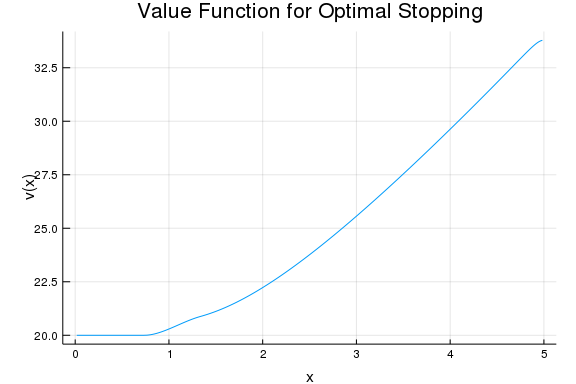## Optimal Stopping Problems, Computational Appendix

Presented by Arnav Sood and Jesse Perla

This notebook is a computational appendix to the earlier notebook on optimal stopping problems, and their formulations as linear complementarity problems (LCPs).

#### Model Setup

As before, we run some setup code

# if you are running locally for the first time, run ] add InstantiateFromURL
using InstantiateFromURL
activate_github_path("QuantEcon/SimpleDifferentialOperators.jl", path = "docs/examples", activate = true)

Updating registry at ~/.julia/registries/General
Updating git-repo https://github.com/JuliaRegistries/General.git
piling project...

using SimpleDifferentialOperators
using Plots, LinearAlgebra, Suppressor, BenchmarkTools


And define a set of model objects

μ = -0.1
σ = 0.1
ρ = 0.05
γ = 0.5
ρ = 0.05
S₀ = 20.0
u(x) = x^γ  # (13)
S(x) = S₀  # (15)
@assert μ <= 0 # otherwise we need to swap the L₁₋bc operator


We can also define the boundary conditions and LCP matrices at this stage

M = 300
x̄ = range(0.0, 5.0, length = (M+2))
x = interiornodes(x̄)
bc = (Reflecting(), Reflecting())  # assume x_t reflects at 0.0 and 5.0
L = I * ρ - μ*L₁₋bc(x̄, bc) - σ^2 / 2 * L₂bc(x̄, bc)  # (2) and (14)

q = -u.(x) + L*S.(x)  # (9)
w(z) = L*z + q; # (10)


#### PATHSolver

This is the first solver we tried. To install it, simply run

# install if required


And to use it

using PATHSolver

lb = zeros(M)
ub = 300*ones(M) # Need to have upper bounds for the z.
code, z, w_val = @btime @suppress solveLCP($w,$lb, $ub) # Solves (12)  5.461 ms (356 allocations: 2.44 MiB)  @assert code == :Solved # otherwise, an error in convergence  We can also try the MCP solver. We expect this to be slower, since we forgo specializations for the linear case. code_, z_, w_val_ = @btime @suppress solveMCP($w, $lb,$ub)  # Solves (12)

23.856 ms (1944 allocations: 33.55 MiB)

@assert code_ == :Solved  # otherwise, an error in convergence


As a sanity check, we can plot

v = z + S.(x)
plot(x, v, title = "Value Function for Optimal Stopping",
legend = false, ylabel = "v(x)", xlabel = "x")Note: As mentioned, for anything other than a rough estimate, the Julia code you're benchmarking should be inside of a function.

#### NLsolve

There is also the NLsolve.jl package, which offers a mcpsolve() method for mixed complementarity problems.

# install if required


And run

using NLsolve

r = @btime @suppress mcpsolve($w,$lb, \$ub,
zeros(300), # initial condition
reformulation = :smooth, # uses a so-called "Fischer function" to smooth out the problem
autodiff = :forward,
inplace = false,
ftol = 1e-12);

203.362 ms (9519 allocations: 51.24 MiB)

@assert converged(r) == true # otherwise, an error in convergence


And the plot

v = r.zero + S.(x)
plot(x, v, title = "Value Function for Optimal Stopping",
legend = false, ylabel = "v(x)", xlabel = "x")#### JuMP (Ipopt)

We can also use JuMP, Julia's DSL for numerical programming.

The solver is the Interior Point OPTimizer (IPOPT).

# install if required


The setup here is a bit different, since we're using the quadratic formulation.

using JuMP, Ipopt

m = Model(with_optimizer(Ipopt.Optimizer, tol=1e-12));
@variable(m, z[1:300]);
@constraint(m, z .>= 0);
@constraint(m, L*z + q .>= 0);
@objective(m, Min, z'*L*z + z'*q);
@suppress optimize!(m);

termination_status(m)

LOCALLY_SOLVED::TerminationStatusCode = 4


And the plot

v = value.(z) + S.(x)
plot(x, v, title = "Value Function for Optimal Stopping",
legend = false, ylabel = "v(x)", xlabel = "x")Note that we didn't compute a benchmark. As optimize!() is in-place (that is, it modifies its arguments), we need to ensure that runs are actually independent.

Here's one approach.

function ipoptFunc()
m = Model(with_optimizer(Ipopt.Optimizer, tol=1e-12));
@variable(m, z[1:300]);
@constraint(m, z .>= 0);
@constraint(m, L*z + q .>= 0);
@objective(m, Min, z'*L*z + z'*q);
@suppress optimize!(m);
end

@benchmark ipoptFunc()

BenchmarkTools.Trial:
memory estimate:  3.61 MiB
allocs estimate:  57669
--------------
minimum time:     103.205 ms (0.00% GC)
median time:      121.977 ms (0.00% GC)
mean time:        123.279 ms (0.91% GC)
maximum time:     138.994 ms (5.32% GC)
--------------
samples:          41
evals/sample:     1


In other words, we're benchmarking the process of building and solving a model from scratch.

Note that if you want more granular performance data, you can use the @benchmark macro as above.

#### JuMP (OSQP)

The flexibility of JuMP is that, after formulating the problem, swapping out solvers is fairly trivial.

Here's the same code, using the Oxford OSQP solver (Operator Splitting for Quadratic Programs).

As you can see, only one line needed to change.

# install if required


And run

using OSQP

m = Model(with_optimizer(OSQP.MathOptInterfaceOSQP.Optimizer))
@variable(m, z[1:300]);
@constraint(m, z .>= 0);
@constraint(m, L*z + q .>= 0);
@objective(m, Min, z'*L*z + z'*q);
@suppress optimize!(m);

@show termination_status(m)

termination_status(m) = ALMOST_OPTIMAL::TerminationStatusCode = 7
ALMOST_OPTIMAL::TerminationStatusCode = 7


And the plot

v = value.(z) + S.(x)
plot(x, v, title = "Value Function for Optimal Stopping",
legend = false, ylabel = "v(x)", xlabel = "x")We can also write benchmarking code as above

function osqpFunc()
m = Model(with_optimizer(OSQP.MathOptInterfaceOSQP.Optimizer))
@variable(m, z[1:300]);
@constraint(m, z .>= 0);
@constraint(m, L*z + q .>= 0);
@objective(m, Min, z'*L*z + z'*q);
@suppress optimize!(m);
end

@benchmark osqpFunc()

BenchmarkTools.Trial:
memory estimate:  4.14 MiB
allocs estimate:  61518
--------------
minimum time:     48.092 ms (0.00% GC)
median time:      55.280 ms (0.00% GC)
mean time:        56.038 ms (2.47% GC)
maximum time:     69.130 ms (0.00% GC)
--------------
samples:          90
evals/sample:     1


#### Conclusions

The outcomes of this notebook are:

• Expository code for solving an LCP-formulated stopping problem using a variety of Julia tools.

• The finding that using code optimized for the linear case leads to noticeable speed gains, and that PATHSolver.jl is a good bet for small problems like this one.

• Note: The size is crucial for this kind of analysis. JuMP (for example) is designed with massive problems in mind, and as such might be doing work that's costly here, but vital for the larger case.